Courses
Courses for Kids
Free study material
Free LIVE classes
More

# Manometer and Type of Manometer for JEELIVE
Join Vedantu’s FREE Mastercalss

## What is The Manometer?

A Manometer is a device that is used for the evaluation of the pressure of a fluid by comparing it with a pre-determined column of standard fluid. In most cases, the standard fluid is mercury. One of the most common types of manometers used by the doctor for measuring blood pressure is a sphygmomanometer. The pressure is expressed in the unit of mm of mercury.

This article gives conceptual knowledge of the manometer. It discusses various topics such as use of a manometer, types of manometer, single column manometer, simple u-tube manometer, inclined manometer, and the manometer diagram. It covers all the mentioned topics briefly with a diagram.

## Types of Manometer

There are different types of manometers used for the measurement of the pressure by the height raised of the liquid column by comparing it with standard liquid. In most of the cases, it was preferred as mercury. There are two categories of manometer which are shown below:

• Simple manometer

• Differential manometer

A Simple manometer is a manometer that is made up of a glass tube having one end connected to a point where the pressure can be measured and the other end of the tube will be open to the atmosphere.

• Piezometer

• U-tube Manometer

• Single column Manometer

A differential manometer is a type of manometer which is used for calculating the difference in pressure between two points in a pipe. There are two types of differential manometer which are shown below:

• U-tube differential manometer

• Inverted U-tube differential manometer

## Piezometer

Piezometer is the simplest form of manometer which is generally known as an open tube manometer. One of the ends of this manometer is connected to the point where the pressure is to be measured and another end of this manometer is open to the atmosphere. The rise in the liquid column will give the pressure at that point.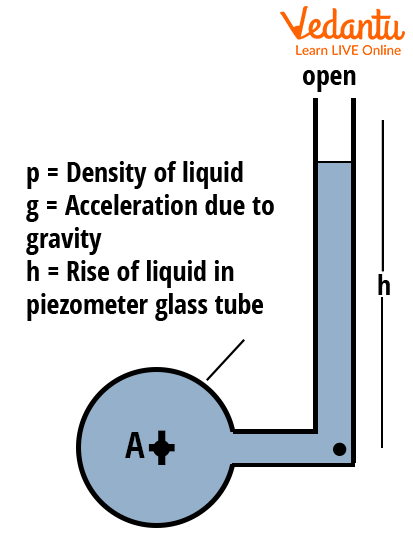A Schematic Diagram of the Piezometer

The pressure at point A, PA will be evaluated with the help of the given formula which is shown below,

• $P_A=\rho\times g\times h$

## U-Tube Manometer

It is a manometer that consists of a glass tube that is bent in a U-shape where one end of the tube is connected to a point where the pressure is to be measured and the other end remains open to the atmosphere.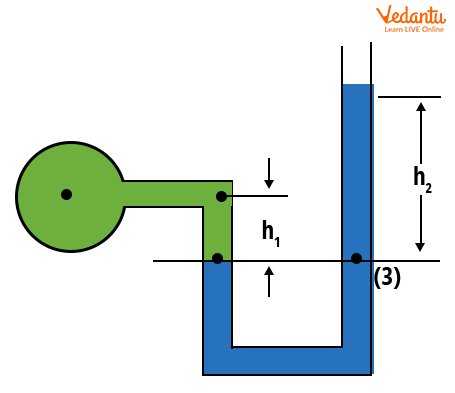A schematic Diagram of the U-Tube Manometer

The gauge pressure at reference level (3) is determined with the help of the given formula

$p=\rho_2 gh_2-\rho_1 gh_1$

Where,

$\rho_1=$The density of low-density liquid

$\rho_2=$The density of high-density liquid

h1= Height of low-density liquid above the reference line

h2= Height of high-density liquid above the reference line

g = Acceleration due to gravity

## Single Column Manometer

It is the modified form of the U-tube manometer in which a reservoir (a section of a large cross-section area nearly 100 times more than the area of the tube) is placed in the manometer.   The presence of the reservoir becomes the reason for the variation in the pressure and the change in height in the liquid column gives the value of pressure. It is of two types which are shown below:

• Vertical single-column manometer

• Inclined single-column manometer

## Vertical Single Column Manometer

In a Vertical single-column manometer, one limb of the manometer consists of a large container-like structure which is termed a reservoir and another limb of the manometer is a simple tube. The height raised in the tube will define the pressure.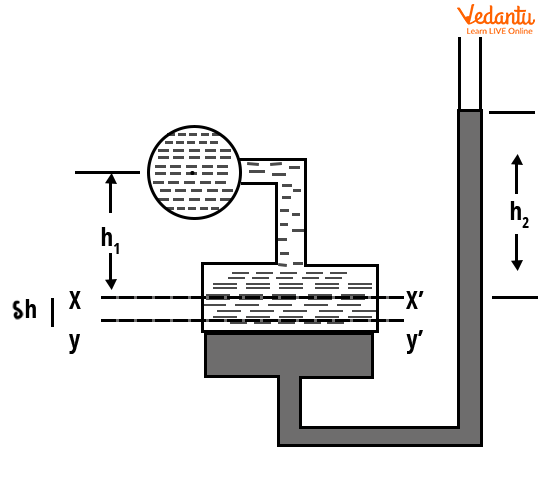A Schematic Representation of a Vertical Single Column Manometer

The formula for determining the magnitude of pressure at the reference line XX’ in a vertical single-column manometer is given by;

$P=\rho_{2} g h_{2}-\rho_{1} g h_{1}$

Where,

$\rho_{1}=$ The density of low-density liquid

$\rho_{2}=$ The density of high-density liquid

g = Acceleration due to gravity

h1= Height of low-density liquid above the reference line XX’

h2= Height of high-density liquid above the reference line XX’

## Inclined Single Column Manometer

In the Inclined single-column manometer, it is just like the previous type of manometer. It has one large section which is termed a reservoir and another limb of the manometer is a simple tube that is inclined to a certain angle. The rise of the fluid in the tube will determine the pressure.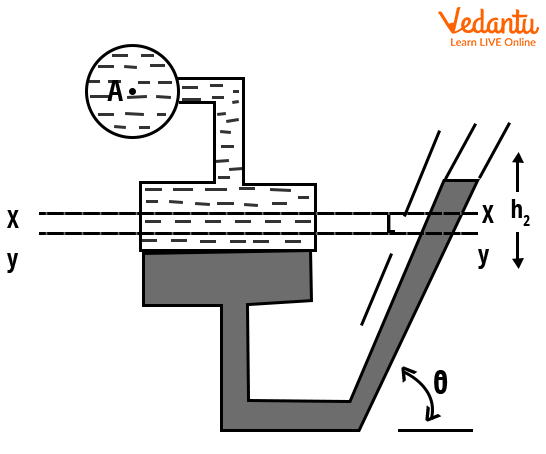A Schematic Representation of Inclined Single Column Manometer

The formula for determining the magnitude of pressure in the inclined single column manometer above the reference line XX is given by;

$P=\rho_{2} g \sin \theta-\rho_{1} g h_{1}$

Where,

$\rho_{1}=$ The density of low-density liquid

$\rho_{2}=$ The density of high-density liquid

h1= Height of low-density liquid above the reference line XX

h2= Height of high-density liquid above the reference line XX

g = Acceleration due to gravity

$\theta=$ Angle of inclination

## U-Tube Differential Manometer

The differential manometer is used to measure the pressure between the two points in a pipe. For this purpose, a differential manometer is used. A U-Tube manometer is a type of differential manometer that consists of two sections that are connected by a U-tube. There is no open end to the atmosphere just like in the previous. In this manometer, the pressure is determined between the two measured points. The change in the height of the fluid with respect to the reference line (or datum line) will give the pressure. The formula for determining the magnitude of pressure in a U-tube differential manometer is given as,

• When both marked points A and B are at a different level, then the difference of pressure between the two points will be given as,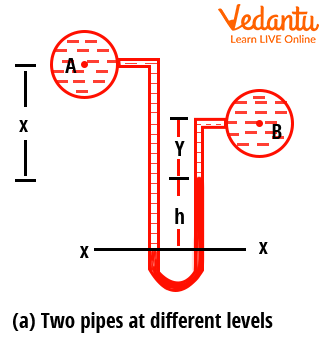A Schematic Representation of a Differential U-Tube Manometer

The pressure between two points A and B in a differential U-tube manometer is determined by the given formula,

$P_{A}-P_{B}=h g\left(\rho_{g}-\rho_{1}\right)+\rho_{2} g y-\rho_{1} g x$

Where,

$\rho_{1}=$ The density of the liquid in section A

$\rho_{2}=$ The density of the liquid in section B

$\rho_{g}=$ The density of high-density liquid

PA = Pressure at the point A

PB = Pressure at the point B

h = Difference of high-density liquid in the right limb of the manometer

g = Acceleration due to gravity

x = Difference between the center of A from the mercury level in the right limb

y = Difference between the center of B from the mercury level in the right limb

• When both marked points are at the same level, then the difference of pressure between two points will be given as,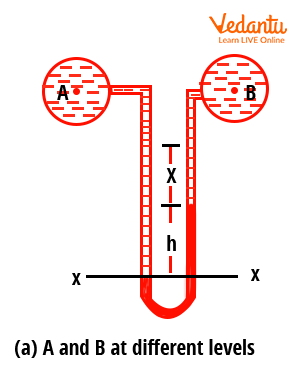A Schematic Representation of Differential U-Tube Manometer

The pressure between two points A and B in a differential U-tube manometer is determined by the given formula,

$P_{A}-P_{B}=g h\left(\rho_{g}-\rho_{1}\right)$

Where,

$\rho_{1}=$ The density of the liquid in section A

$\rho_{g}=$ The density of high-density liquid

PA = Pressure at the point A

PB = Pressure at the point B

h = Difference of high-density liquid in a right limb of a manometer

g =Acceleration due to gravity

## Inverted U-Tube Differential Manometer

An inverted U-tube manometer is a type of differential manometer that consists of two sections that are connected by an inverted U-tube. There is no open end to the atmosphere just like in the previous. In this manometer, the pressure is determined between the two measured points. The change in the height of the fluid with respect to the reference line (or datum line) will give the pressure.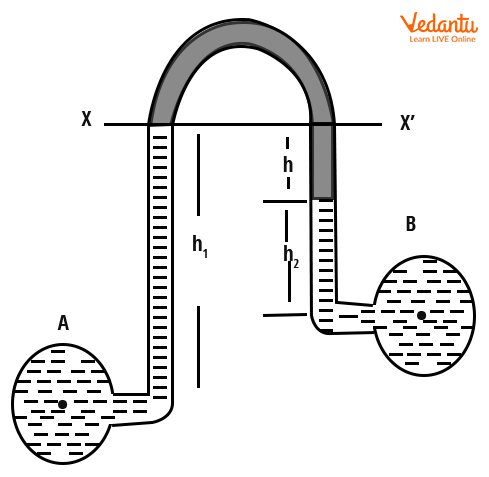A Schematic Representation of an Inverted U-Tube Manometer

The formula for determining the magnitude of pressure in an inverted U-tube differential manometer is given,

$P_{A}-P_{B}=h_{1} g \rho_{1}-\rho_{2} g h_{2}-\rho_{l} g h$

Where,

$\rho_{1}=$ The density of the liquid in section A

$\rho_{2}=$ The density of the liquid in section B

$\rho_{l}=$ The density of high-density liquid

PA= Pressure at the point A

PB= Pressure at the point B

h = Difference between low-density liquid

h1= Height of liquid in left limb below the reference line XX

h2= Height of liquid in the right limb

g = Acceleration due to gravity

## Conclusion

This article gives detailed information about the concept of a manometer and its types. The article starts with the clarification of the first query, i.e. what is a manometer. This article explained the types of manometers along with the manometer diagram and formula for determining the magnitude of the pressure. It has also explained the differential manometer and its types with a diagram. This article covers the manometer concept from a diagram point of view for a better understanding of the concept.

Last updated date: 22nd Sep 2023
Total views: 136.8k
Views today: 1.36k

## FAQs on Manometer and Type of Manometer for JEE

1. What is the limitation of the manometer?

It is known that a manometer is used to measure the pressure at any point with the help of a fluid system. This system has various limitations which makes it difficult to use. This concept of a manometer requires a heavy and bulky apparatus which makes it difficult to handle. There can be an error in the measurement of pressure due to the condensation as there is the fluid used in it. The manometer has very negative dynamic results. This device also requires a leveling arrangement for pressure measurement.

2. What is the requirement of the large reservoir in the single-column manometer?

The large reservoir is required in the single column manometer because it directly allows the observer to evaluate the pressure by raising a corresponding height in the tube of the manometer. By introducing a large sectional cross-section area of the reservoir, there is a certain pressure difference that occurred due to the change in the area of the tube and the reservoir. This is the reason for which the large reservoir is introduced in the single-column manometer. This setup makes the evaluation of pressure easy for the observer.

3. What is the weightage of this topic in the JEE exam?

The weightage of the current topic titled as manometer is 3%. This concept is introduced in the section on the mechanics of fluids which has a large variety of important concepts for the JEE examination. Nearly 1-2 questions can be expected in the given competitive examination of JEE. In this chapter, it is required to keep a check on every topic and practice a maximum number of questions for better performance in this section. Hence, it is required to give importance to this chapter from JEE's point of view.